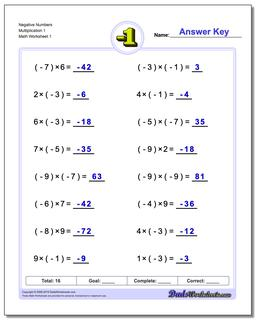One Dad. Four daughters. 9,341 worksheets... and counting!# Negative Numbers Multiplication 1

PropertyValue
DescriptionNegative Numbers Multiplication 1: The worksheets in this section introduce negative numbers integers in multiplication and division math problems. All problems deal with smaller integers that can be solved without multi-digit multiplication or long division.
Resource TypeWorksheet